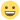## multi level logistic regression ICC

Discuss statistics related things

## multi level logistic regression ICC

Hello there,

Can anyone please share with me, where do i find the ICC in the null model or model 1 of a multi level logistic regression?

Normally, in multi leveling models, the null model will show the ICC, indicating if it is justified to continue with multi level model. But in Multi level logistic regression, it does not show directly the ICC. so i do not know if continuing with multi level model is justified considering my data set.

Please helpthank you in advance. warm regards,

Enrico Mendoza
enricocmendoza

Posts: 10
Joined: Fri Oct 23, 2020 9:12 am

## Re: multi level logistic regression ICC

In mixed logistic regression, the error variance is set to 1, so the ICC is simply the variance of the intercept divided by 1+the variance of the intercept. Thus, if there's variance in the intercepts, you are justified to run the multilevel logistic regression.

I'd add, however, that just simply looking at the ICC of the null model is not necessarily a good strategy to disregard a mixed model when the ICC is zero (o very small). You can have, in fact, models with huge variances of the slopes and no variances of the intercepts. Thus, a better strategy is to estimate the model with all possible random effects, and check if any of the random effects have variance greater than zero.mcfanda@gmail.com

Posts: 245
Joined: Thu Mar 23, 2017 9:24 pm

## Re: multi level logistic regression ICC

thank you so much for this reply.

God bless always.
enricocmendoza

Posts: 10
Joined: Fri Oct 23, 2020 9:12 am

Return to Statistics